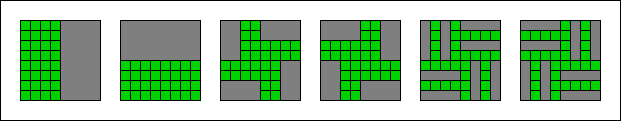## Minimum Area Polyomino Venn Diagrams

Bette Bultena, Department of Computer Science, University of Victoria, Canada.
Matthew Klimesh, Jet Propulsion Laboratory, Pasadena, California, USA.
Frank Ruskey, Department of Computer Science, University of Victoria, Canada.

### Abstract:

Polyomino Venn (or polyVenn) diagrams are Venn diagrams whose curves are the perimeters of orthogonal polyominoes drawn on the integer lattice. Minimum area polyVenn diagrams are those in which each of the 2n regions, in a diagram of n polyominoes, consists of exactly one unit square. We construct minimum area polyVenn diagrams in bounding rectangles of size 2r X 2c whenever r, c > 2. Our construction is inductive, and depends on two "expansion" results. First, a minimum area polyVenn diagram in a 2r X 2c rectangle can be expanded to produce another that fits into a 2r+1 X 2c+1 rectangle. Secondly, when r = 2, it can also be expanded to produce a polyVenn diagram in a 2r X 2c+3 bounding rectangle. Finally, we construct polyVenn diagrams in bounding rectangles of size 2n/2 -1 X 2n/2 +1 if n is even, but where the empty set is not represented as a unit square.

• The pdf file.
• Appears in Journal of Computational Geometry, (3)1 (2012) 154-167.
• Mark Thompson's page on Venn Polyominos is what got us started on this research. Unfortunately, his page seems to no longer be active, but we have reproduced it here using the "Wayback Machine".
• For more on Venn diagrams check out the Survey of Venn Diagrams, Dynamic Survey #5 at the Electronic Journal of Combinatorics.

Selected papers that refer to this one:
• TBA.

## Six and Seven Set PolyVenn DiagramsBack to list of publications.